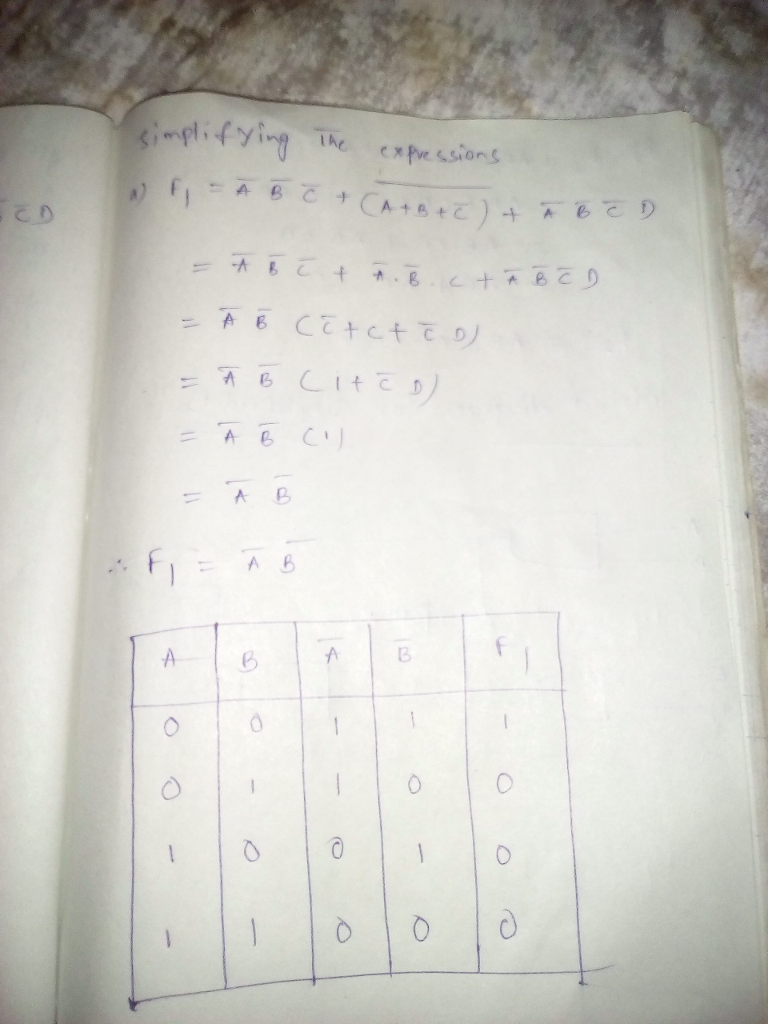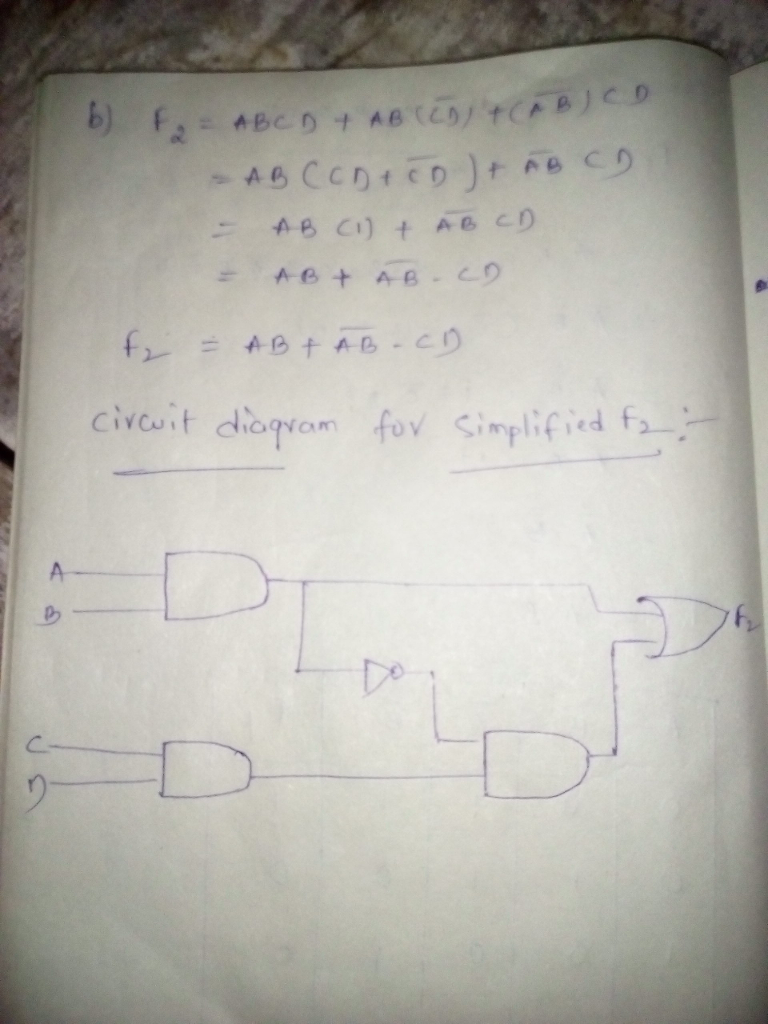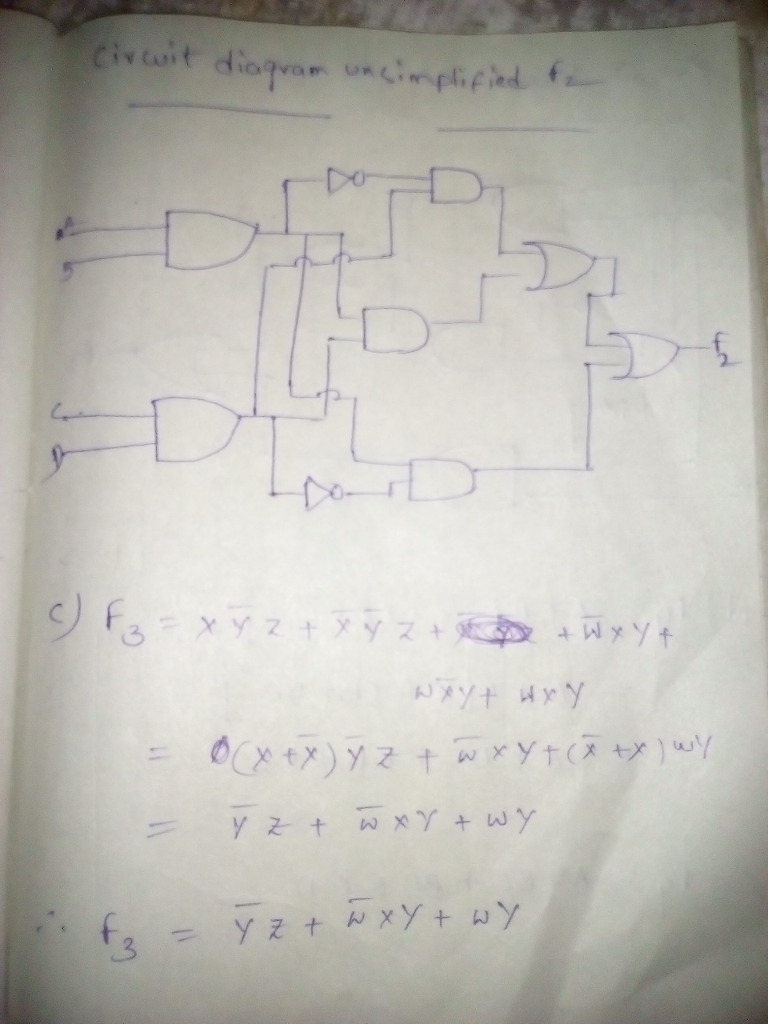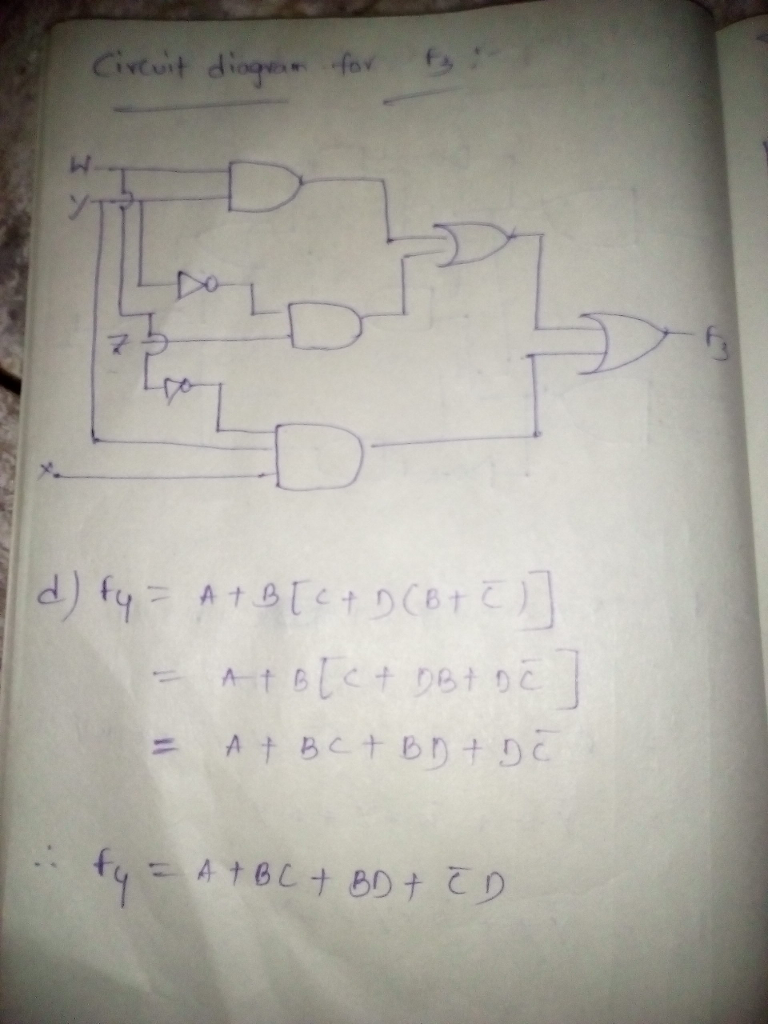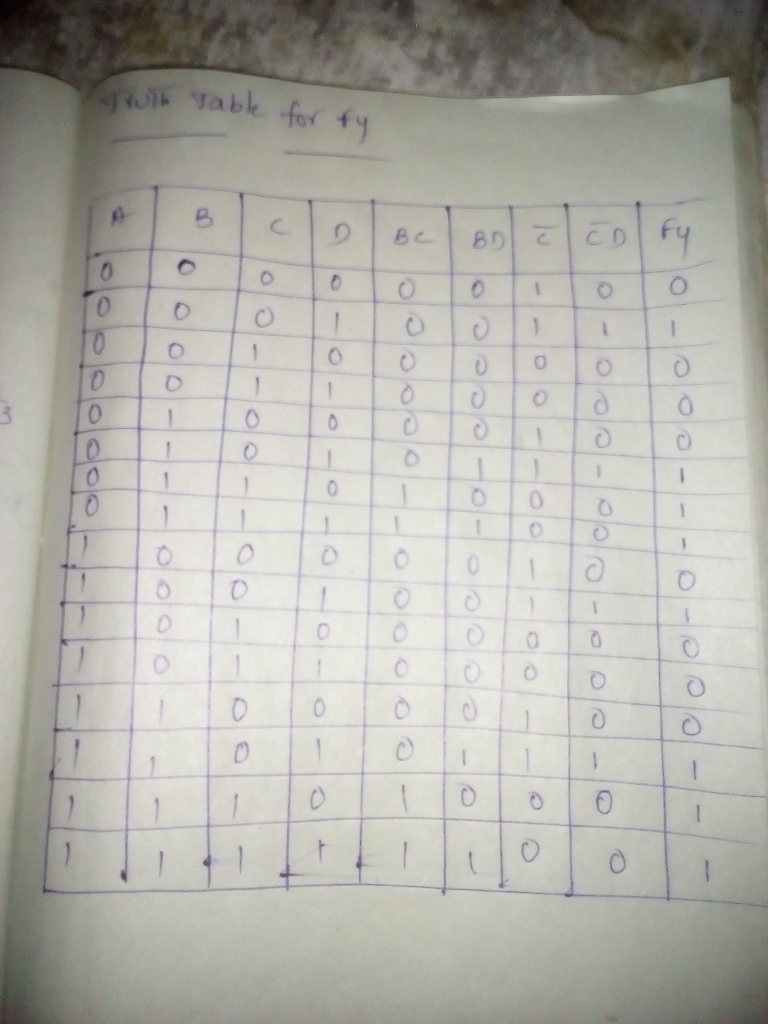# Homework Solution: Using Boolean algebra, simplify the following expressions. F_1 = A bar B bar C bar + (A + B + C bar) wholebar + A bar…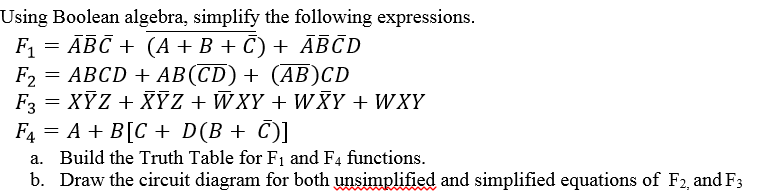Using Boolean algebra, simplify the following expressions. F_1 = A bar B bar C bar + (A + B + C bar) wholebar + A bar B bar C bar D F_2 = ABCD + AB(CD wholebar) + (AB wholebar)CD F_3 = XY bar Z + X bar Y bar Z + W bar XY + W X bar Y + WXY F_4 = A +B[C + D(B + C bar)] a. Build the Truth Table for F_1 and F_4 functions. b. Draw the circuit diagram for both unsimplified and simplified equations of F_2, andF_3.